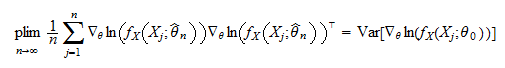StatLect

Maximum likelihood - Covariance matrix estimation

In the lecture entitled Maximum likelihood we have demonstrated that, under certain assumptions, the distribution of the maximum likelihood estimatorof a vector of parameterscan be approximated by a multivariate normal distribution with meanand covariance matrixwhereis the log-likelihood of one observation from the sample, evaluated at the true parameter, and the gradientis the vector of first derivatives of the log-likelihood.

Becauseis unknown, also this covariance matrix is unknown. Here we discuss methods to consistently estimate it.

We make the same assumptions made in the aforementioned lecture. So, for example,whereare the realizations of the firstterms of an IID sequence. Under these assumptions we also have that the information equality holds, so thatwhere the Hessian matrixis the matrix of second-order partial derivatives of the log-likelihood function.Outer product of gradients (OPG) estimate

The first estimate of the asymptotic covariance matrixis called outer product of gradients (OPG) estimate and it is computed asIt takes its name from the fact that the gradientis a column vector, its transpose is a row vector, and the product between a column and a row is called outer product.

Provided some regularity conditions are satisfied, the OPG estimatoris a consistent estimator of, that is, it converges in probability to.

Proof

We provide only a sketch of the proof and we refer the reader to Newey and McFadden (1994) for a more rigorous exposition. Provided some regularity conditions are satisfied (see the source just cited), we have the following equality between probability limits:wherehas been replaced bybecause, being a consistent estimator, it converges in probability to. Because the sample is IID, by the Law of Large Numbers we have thatNow, the formula for the covariance matrix (see the lecture entitled Covariance matrix) yieldsBut the expected value of the gradient evaluated atis, so thatThus,Because matrix inversion is continuous, by the Continuous Mapping theorem we havewhich is exactly the result we needed to prove.

Hessian estimate

The second estimate of the asymptotic covariance matrixis called Hessian estimate and it is computed asUnder some regularity conditions, the Hessian estimatoris also a consistent estimator of.

Proof

Again, we do not provide an entirely rigorous proof (for which you can see Newey and McFadden - 1994) and we only a sketch the main steps. First of all, under some regularity conditions, we have thatwherehas been replaced bybecause, being a consistent estimator, it converges in probability to. Now, since the sample is IID, by the Law of Large Numbers we have thatBy the information equality, we haveTherefore,Because matrix inversion is continuous, by the Continuous Mapping theorem we havewhich is what we needed to prove.

Sandwich estimate

The third estimate of the asymptotic covariance matrixis called Sandwich estimate and it is computed aswhereis the OPG estimate andis the Hessian estimate.

Also the Sandwich estimatoris a consistent estimator of.

Proof

This is again a consequence of the Continuous Mapping theorem:where the last equality follows from the consistency of the OPG and Hessian estimators.

References

Newey, W. K. and D. McFadden (1994) "Chapter 35: Large sample estimation and hypothesis testing", in Handbook of Econometrics, Elsevier.

The book

Most of the learning materials found on this website are now available in a traditional textbook format.

Glossary entries
Share